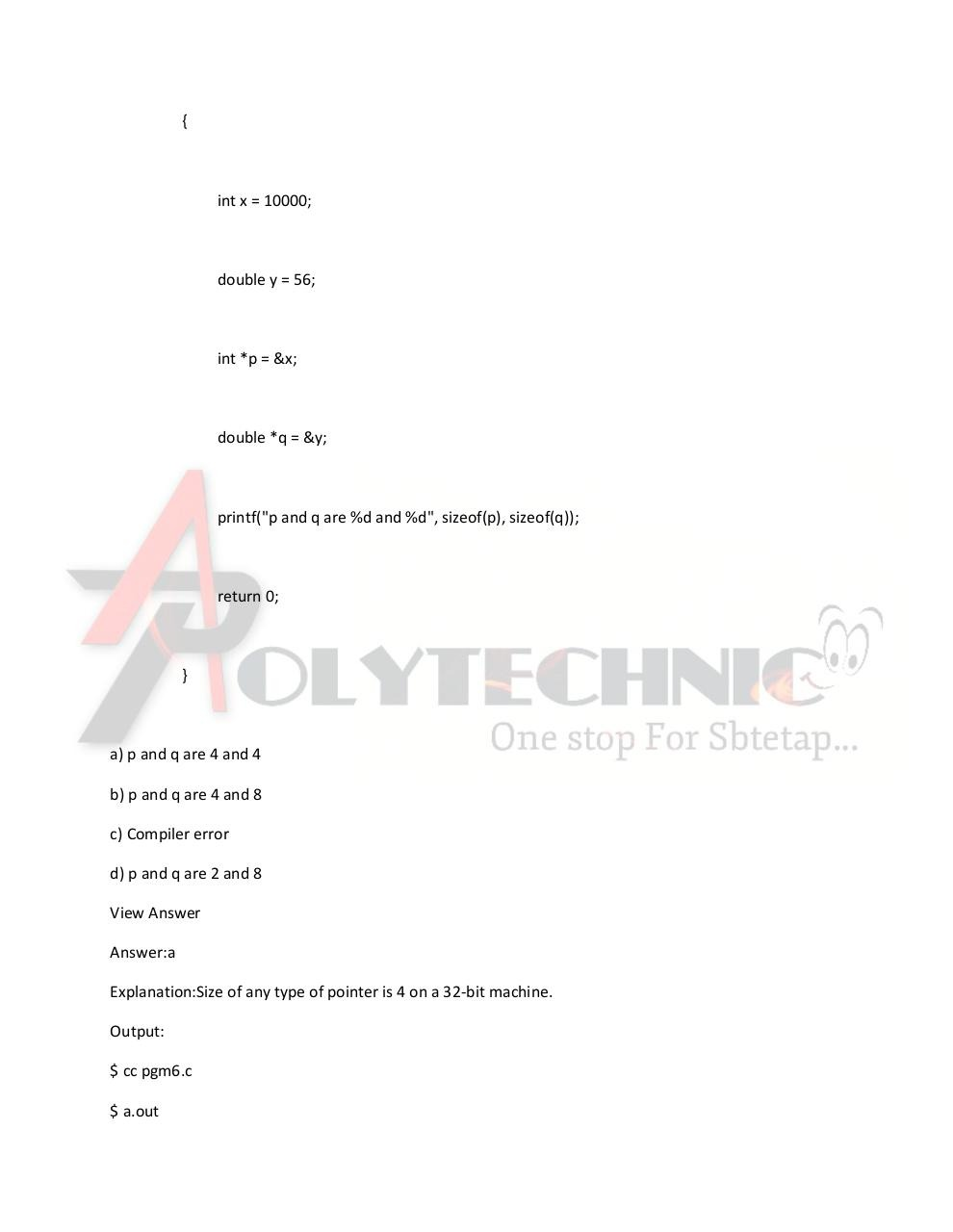# Data Types and Sizes â€“ 2.Image.Marked.pdfPage 1 2 3 4 5 6 7 8

#### Text preview

{

int x = 10000;

double y = 56;

int *p = &amp;x;

double *q = &amp;y;

printf(&quot;p and q are %d and %d&quot;, sizeof(p), sizeof(q));

return 0;

}

a) p and q are 4 and 4
b) p and q are 4 and 8
c) Compiler error
d) p and q are 2 and 8